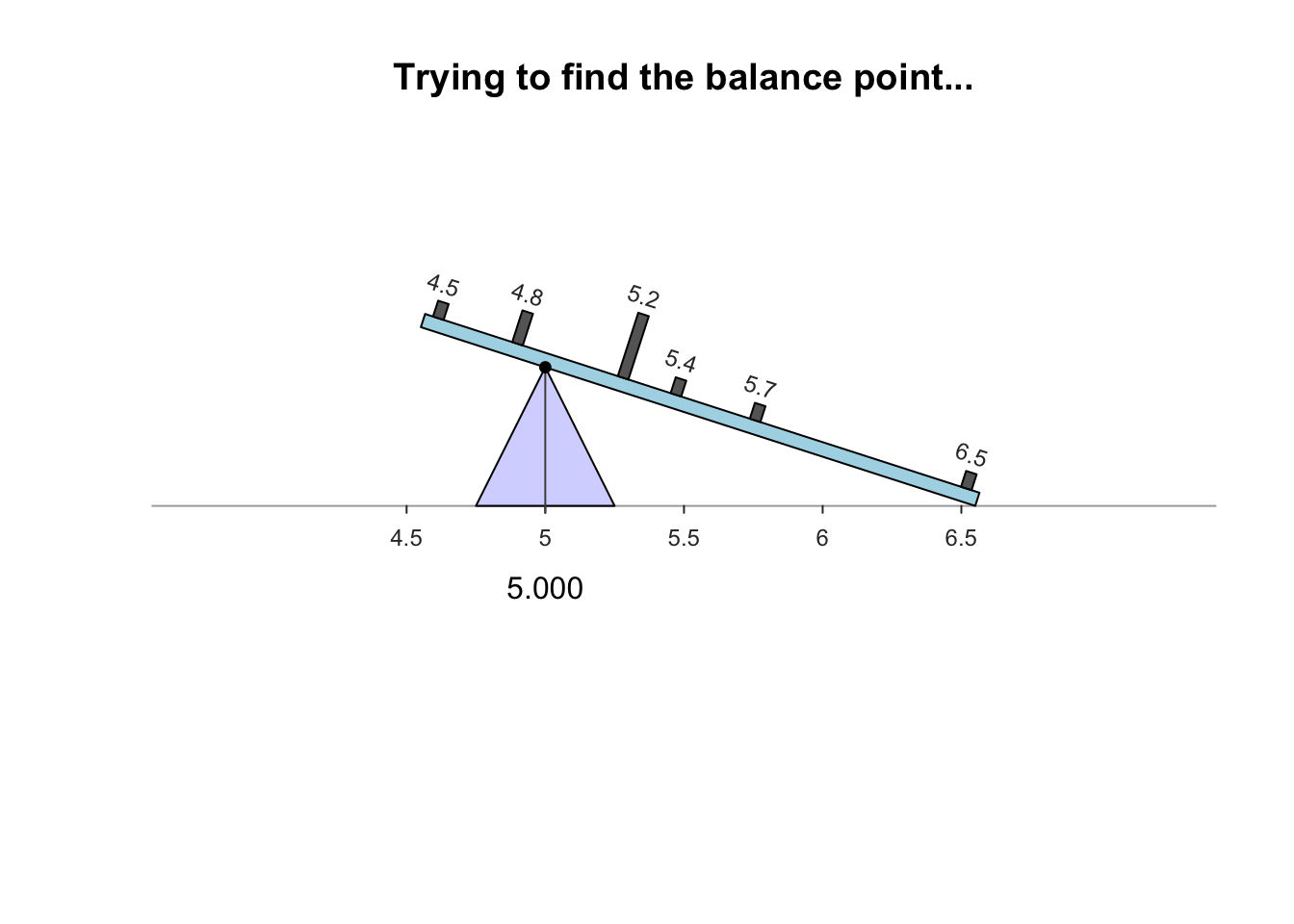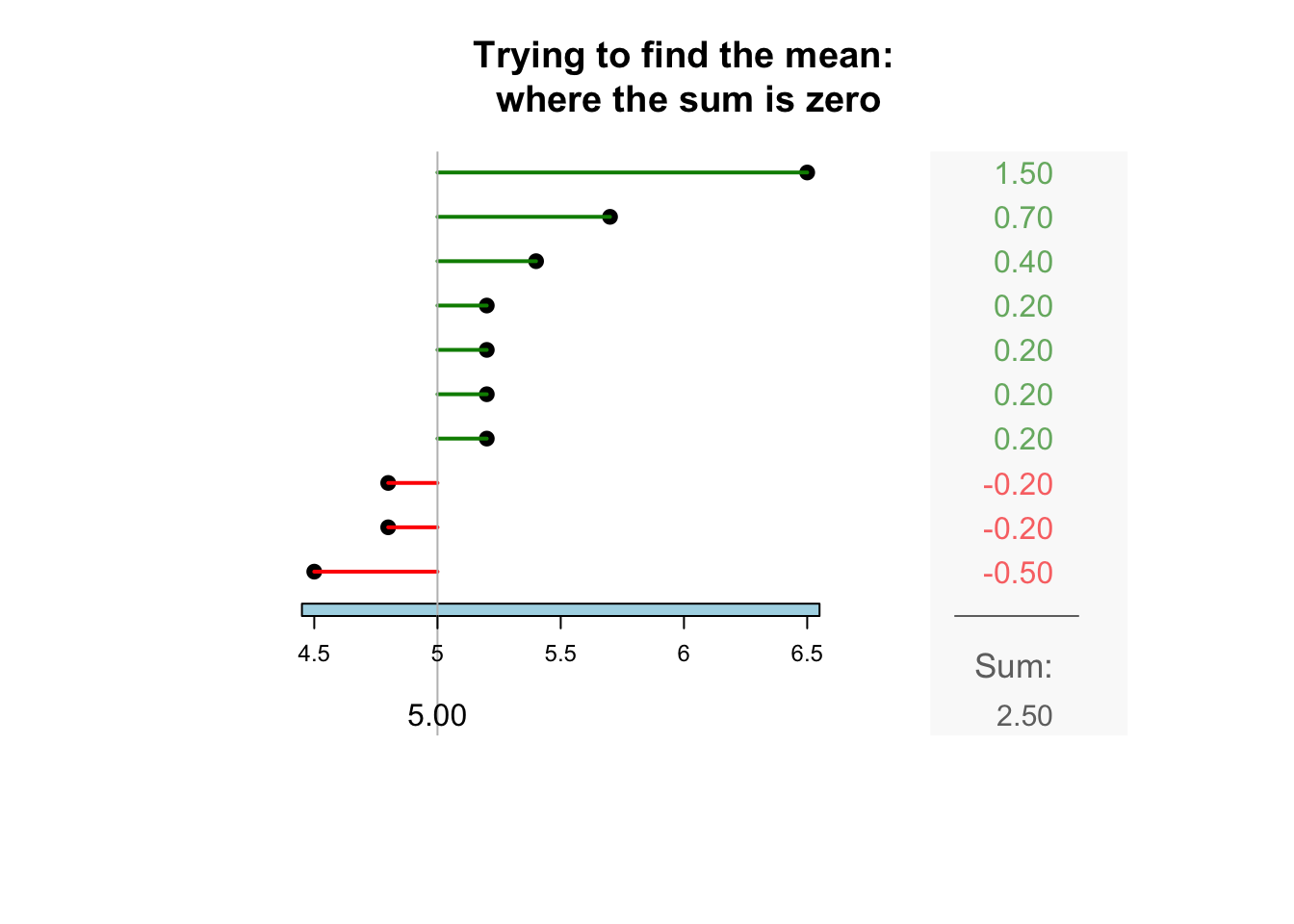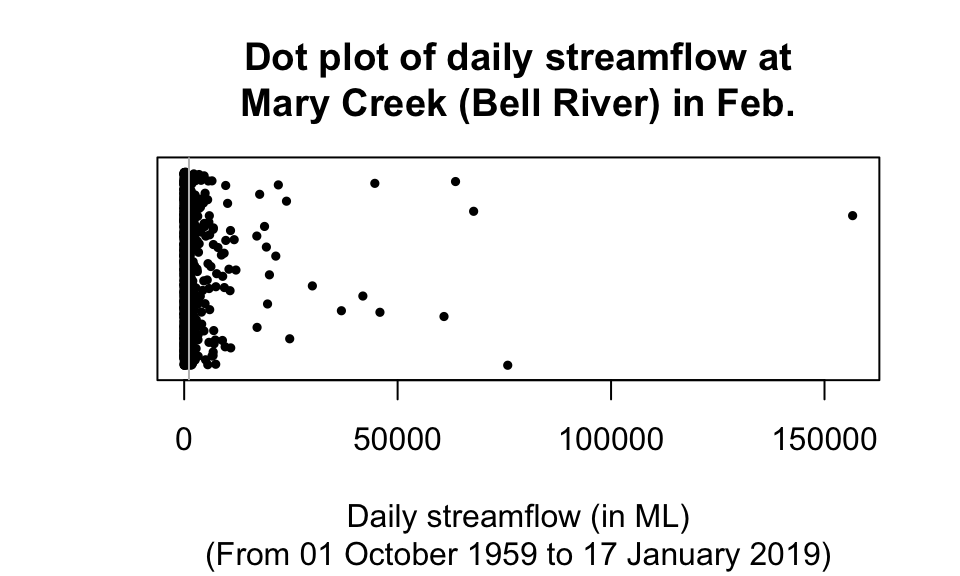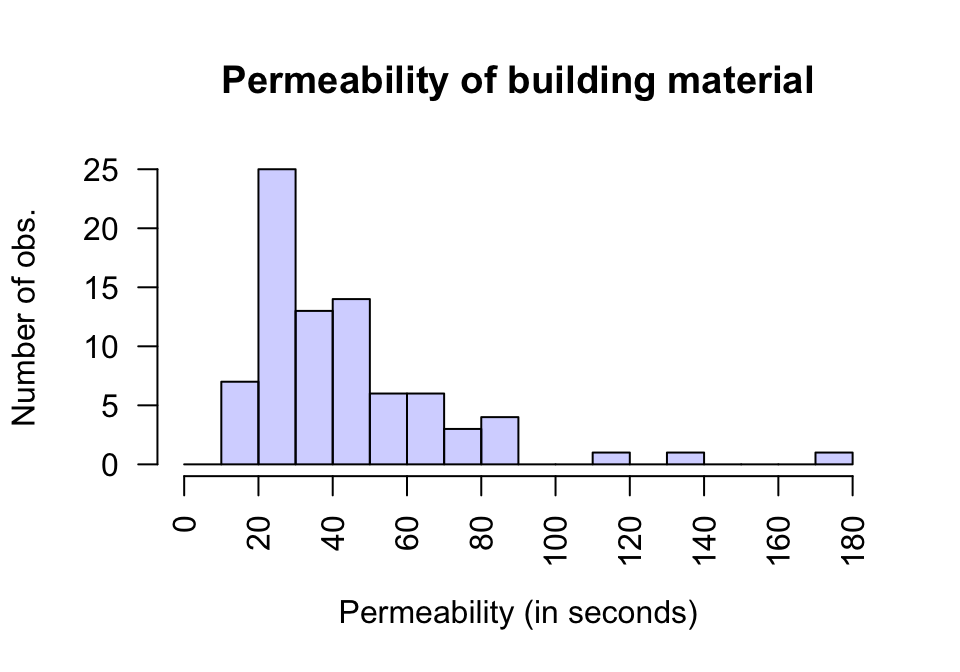## 13.2 Computing the average valueThe average (or location, or centre, or typical value) for quantitative sample data can be described in many ways; the two most common ways are:

In both cases, the population parameter is estimated by a sample statistic. Understanding whether to use the mean or median is important.

The word ‘average’ can refer to either mean or median (or other measures of centre too). Use the precise terms ‘mean’ or ‘median,’ rather than ‘average,’ when necessary!Think 13.1 (Difference between averages) Consider the daily river flow volume (called ‘streamflow’) at the Mary River from 01 October 1959 to 17 January 2019, summarised by month in Table 13.1 (from Queensland DNRM).

The ‘average’ daily streamflow in February could be quoted using either the mean or the median; but the two give very different values for the ‘average’:

• the mean daily flow is 1123.2ML.
• the median daily flow is 146.1ML.
These two common ways of measuring the same thing (the ‘average’ daily streamflow in February) give very different answers. Why? Which is the best ‘average’ to use? To decide, both measures of average will need to be studied.
TABLE 13.1: The daily streamflow at Mary River (Bellbird Creek), in ML, from 01 October 1959 to 17 January 2019; average for each month
Month Mean Median
Jan 849.3 71.3
Feb 1123.2 146.1
Mar 793.9 194.9
Apr 622.5 141.7
May 348.4 118.4
Jun 378.7 83.6
Jul 259.3 68.8
Aug 108.6 55.5
Sep 100.9 48.0
Oct 151.2 37.6
Nov 186.6 45.3
Dec 330.8 64.1

### 13.2.1 Computing the average: The mean

The mean of the population is denoted by $$\mu$$, and its value is almost always unknown.

Instead, the mean of the population is estimated by the mean of the sample, which is denoted by $$\bar{x}$$ (an $$x$$ with a line above it). In this context, the unknown parameter is $$\mu$$, and the statistic is $$\bar{x}$$. The sample mean is used to estimate the population mean.

The Greek letter $$\mu$$ is pronounced ‘myoo,’ as in music.

The symbol $$\bar{x}$$ is pronounced ‘ex-bar.’Example 13.2 (A small data set to work with) To demonstrate ideas, consider a small data set for answering this descriptive RQ:

For mature Jersey cows, what is the average percentage butterfat in their milk?

The population is ‘milk from Jersey cows,’ and an estimate of the population mean percentage butterfat is sought. The population mean is denoted by $$\mu$$.

Clearly, milk from every Jersey cow cannot be studied; a sample is studied : The unknown population mean is estimated using the sample mean ($$\bar{x}$$). Measurements were taken from milk from 10 cows, in percentages (Table 13.2).
TABLE 13.2: The butterfat percentage from a sample of milk from 10 Jersey cows
Butterfat percentages
4.8 5.2 5.2 5.4 5.2
6.5 4.5 5.7 4.8 5.2

The sample mean is what people usually think of as the ‘average.’ The sample mean is actually the ‘balance point’ of the observations. The animation below shows how the mean acts as the balance point. Alternatively, the mean is the value such that the positive and negative distances of the observations from the mean add to zero , as shown in the animation below. Both of these explanations seem reasonable for identifying the ‘average’ of the data.Definition 13.3 (Mean) The mean is one way to measure the ‘average’ value of quantitative data. The arithmetic mean can be considered as the ‘balance point’ of the data, or the value such that the positive and negative distances from the mean add to zero.

To find the value of the sample mean:

• Add (shown using the symbol $$\sum$$) all the observations (denoted by $$x$$); then
• Divide by the number of observations (denoted by $$n$$).

In symbols: $\bar{x} = \frac{\sum x}{n}.$ This means to add up (indicated by $$\sum$$) the observations (denoted by $$x$$), then divide by the size of the sample (denoted by $$n$$).Example 13.3 (Computing a sample mean) For data for the Jersey cow data (Example 13.2), an estimate of the population mean percentage butterfat is found using the sample information: sum all $$n=10$$ observations and divide by $$n$$: \begin{align*} \overline{x} &= \frac{\sum x}{n} = \frac{4.8 + 6.5 + \cdots + 5.2}{10}\\ &= \frac{52.5}{10} = 5.25. \end{align*} The sample mean, the best estimate of the population mean, is 5.25 percent.
Usually, software (such as jamovi or SPSS) or a calculator (in Statistics Mode) will be used to compute the sample mean. However, knowing how these quantities are computed is important.
Think 13.2 (Mean) For the butterfat data (Table 13.2), what is the value of $$\mu$$, the population mean?
We do not know! We know the value of the sample mean, but not the population mean. We only have an estimate of the value of the population mean.
Think 13.3 (Estimating a mean) A study of eyes aimed to estimate the average thickness of eyes affected by glaucoma. The collected data (in microns) are shown in Table 13.3. Estimate the population mean corneal thickness.
TABLE 13.3: The thickness of the cornea (in microns) in eyes affected by glaucoma
Corneal thicknesses
484 492 436 464
478 444 398 476

Software and calculators often produce numerical answers to many decimal places, some of which may not be meaningful or useful. A useful rule-of-thumb is to round to one or two more significant figures than the original data.

For example, the butterfat data are given to one decimal place. The sample mean weight can be given to two decimal places: $$\bar{x}=5.25$$%.

### 13.2.2 Computing the average: The median

The median is a value separating the larger half of the data from the smaller half of the data. In a data set with $$n$$ values, the median is ordered observation number $$\displaystyle \frac{n+1}{2}$$. The median is:

• not equal to $$\displaystyle \frac{n+1}{2}$$.
• not halfway between the minimum and maximum values in the data.

Most calculators cannot find the median.

The median has no commonly-used symbol.
Definition 13.4 (Median) The median is one way to measure the ‘average’ value of some data. The median is a value such that half the values are larger than the median, and half the values are smaller than the median.Example 13.4 (Find a sample median) To find the sample median for the Jersey cow data (Example 13.2), first arrange the data in numerical order (Table 13.4). The median separates the larger 5 numbers from the smaller 5 numbers. With $$n=10$$ observations, the median is the ordered observation located between the fifth and sixth observations (i.e., at position $$(10 + 1)/2 = 5.5$$; the median itself is not 5.5). So the sample median is between $$5.2$$ (ordered observation five) and $$5.2$$ (ordered observation six): the sample median is $$5.20$$ percent.
TABLE 13.4: The butterfat percentage from a sample of milk from 10 Jersey cows, in increasing order
Butterfat percentages
4.5 4.8 4.8 5.2 5.2
5.2 5.2 5.4 5.7 6.5
Think 13.4 (Median) For the butterfat data (Table 13.2), what is the population median?
We do not know! We know the value of the sample median, but not the population median. We only have an estimate of the value of the population median.

Think 13.5 (Medians) A study of eyes aimed to estimate the average thickness of eyes affected by glaucoma.

Using the collected data (Table 13.3), estimate the population median corneal thickness. What is the population median?

With $$n = 8$$ observations, the median is ordered observation number $$(8 + 1)/2 = 4.5$$, halfway between ordered observation numbers 4 and 5. After sorting into increasing order, the two middle numbers (the 4th and 5th) are 464 and 476. The median could be any number between 464 and 476, but the usual answer would be that the median is $$(464 + 476)/2 = 470$$.

The sample median is 470 microns; the value of the population median remains unknown.

To clarify:

• If the sample size $$n$$ is odd, the median is the middle number when the observations are ordered.
• If the sample size $$n$$ is even (such as in Think 13.5), the median is halfway between the two middle numbers, when the observations are ordered.

Some software uses different rules when $$n$$ is even.

### 13.2.3 Which average to use?

Consider again estimating the average daily streamflow at the Mary River (Bellbird Creek) during February (Table 13.1): The mean daily streamflow is 1123.2ML, and the median daily streamflow is 146.1ML. Which is the ‘best’ average to use?

A dot chart of the daily stream flow (Fig. 13.2) shows that the data are very highly right-skewed, with many very large outliers: the maximum value is 156586.4ML, more than one hundred times larger than the mean of 1123.2ML). In fact, about 86% of the observations are less than the mean. In contrast, about 50% the values are less than the median (by definition). For these data, the mean is hardly a central value…FIGURE 13.2: A dot plot of the daily streamflow at Mary River from 1960 to 2017, for February. The vertical grey line is the mean value. Many large outliers exist, so the data near zero are all squashed together

The streamflow data are very highly skewed (to the right), which is important and relevant:

• Means are best used for approximately symmetric data: the mean is influenced by outliers and skewness.
• Medians are best used for data that are skewed or contain outliers: the median is not influenced by outliers and skewness.

Means tend to be too large if the data contains large outliers or severe right skewness, and too small if the data contains small outliers or severe left skewness.

For the Mary River data, the large outliers—and the fact that they are so extreme and abundant—result in the mean being substantially influenced by the outliers, which explains why the mean is much larger than the median. The median is the better measure of average for these data.

The mean is generally used if possible (for practical and mathematical reasons), and is the most commonly-used measure of location. However, the mean is influenced by outliers and skewness; the median is not influenced by outliers and skewness. The mean and median are similar in approximately symmetric distributions. Sometimes, quoting both the mean and the median may be appropriate.

Think 13.6 (Which average to use) An engineering study was studying a new building material to determine the average permeability time.

The time (in seconds) taken for water to permeate $$n=81$$ pieces of material. Using a histogram of the data (Fig. 13.3), estimate the value of the population mean and median. Which would be best to use (for example, to quote an average permeability time on a specification sheet)?FIGURE 13.3: A histogram of the permeability of a type of building material

### References

Ehlers N. On corneal thickness and intraocular pressure. II: A clinical study on the thickness of the corneal stroma in glaucomatous eyes. Acta ophthalmologica. Wiley Online Library; 1970;48(6):1107–12.
Hald A. Statistical theory with engineering applications. New York: John Wiley; Sons; 1952.
Hand DJ, Daly F, Lunn AD, McConway KY, Ostrowski E. A handbook of small data sets. London: Chapman; Hall; 1996.
Sokal RR, Rohlf FJ. Biometry: The principles and practice of statistics in biological research. Third. New York: W. H. Freeman; Company; 1995.